# Cross product

Cross product is a binary operation on two vectors in three-dimensional space. It results in a vector which is perpendicular to both vectors. Vector product of two vectors a and b is denoted by a × b. Its resultant vector is perpendicular to a and b. Vector products are also called a cross product. Cross product of two vectors will give the resultant as a vector and it is calculated using the Right-hand Rule. In this article, we are going to discuss the cross product of two vectors, formulas, properties and examples in detail.

## Cross Product Definition

Vector product or cross product of two vectors $\vec{A}$ and $\vec{B}$ is denoted by A×B and its resultant vector is perpendicular to the vectors $\vec{A}$ and $\vec{B}$. The cross product is mostly used to determine the vector which is perpendicular to the plane surface spanned by two vectors whereas the dot product is used to find the angle between two vectors or the length of the vector. The cross product of two vectors say a × b, is another vector that is at right angles to the both, and it absolutely happens in the three-dimensions.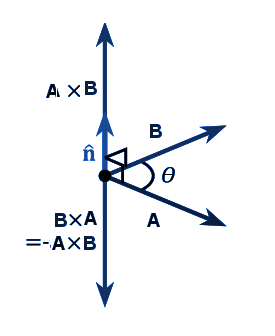## Cross product Formula

If θ is the angle between the given vectors, then the formula for the cross product of vectors is given by

A×B=AB sinθ

Cross Product of Two vectors

Cross product of two vectors is indicated as: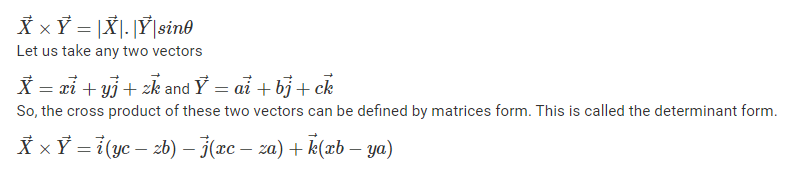## Cross product Properties

To find the cross product of two vectors, we can use properties.  The properties such as anti-commutative property, zero vector property plays an important role in finding the cross product of two vectors. Apart from these properties, some other properties are Jacobi property, distributive property. The properties of cross-product are given below: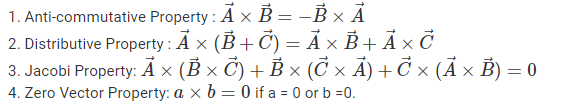## Right-hand Rule Cross Product

The direction of the unit vector can be found with the help of the right-hand rule. In this rule, we can stretch our right hand in a way that the index finger of the right hand in the direction of the first vector and the middle finger is in the direction of the second vector. Then, the thumb of the right hand indicates the direction or unit vector n. With the help of the right-hand rule, we can easily show that the cross product of vectors is not commutative. If we have two vectors A and B, then the diagram for the right-hand rule is as follows: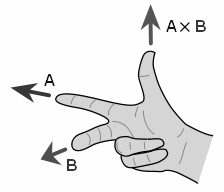## Cross Product of Perpendicular Vectors

Cross product of two vectors is equal to the product of their magnitude, which represents the area of a rectangle with sides X and Y. If two vectors are perpendicular to each other, then the cross product formula becomes:

Θ = 90 degrees

Therefore, sin 90° = 1

So,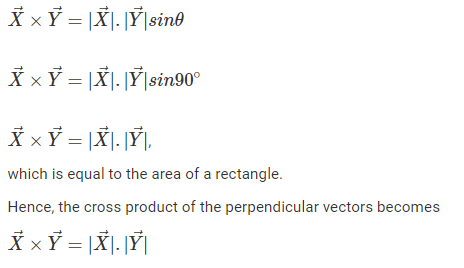## Cross Product of Parallel vectors

The cross product of two vectors are zero vectors if both the vectors are parallel or opposite to each other. Conversely, if two vectors are parallel or opposite to each other, then their product is a zero vector. Two vectors have the same sense of direction.

Θ = 90 degrees

Therefore, sin 0° = 0

Therefore, sin 90° = 1

So,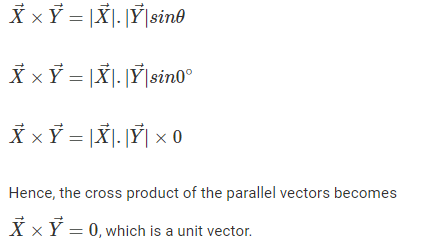### Magnitude of Cross Product

Let us assume two vectors, $\vec{A}= A_{x}+ A_{y}+ A_{z}$ and $\vec{B}= B_{x}+ B_{y}+ B_{z}$, then the magnitude of two vectors are given by the formula,

$A = \sqrt{A_{x}^{2} + A_{y}^{2}+ A_{z}^{2}}$, and

$B = \sqrt{B_{x}^{2} + B_{y}^{2}+ B_{z}^{2}}$

Hence, the magnitude of the cross product of two vectors are given by the formula,

$|A\times B|= |A| |B| sin\theta$

### Cross Product Example

Example:

Find the cross product of the given two vectors: $\vec{X}= 5\vec{i} + 6\vec{j} + 2\vec{k}$ and $\vec{Y}= \vec{i} + \vec{j} + \vec{k}$

Solution:

Given:

$\vec{X}= 5\vec{i} + 6\vec{j} + 2\vec{k} \\ \vec{Y}= \vec{i} + \vec{j} + \vec{k}$

To find the cross product of two vectors, we have to write the given vectors in determinant form. Using the determinant form, we can find the cross product of two vectors

$\vec{X}\times \vec{Y}= (6-2)\vec{i}-(5-2)\vec{j}+ (5-6)\vec{k}$

Therefore, $\vec{X}\times \vec{Y}= 4\vec{i}-3\vec{j}- \vec{k}$

Visit BYJU’S – The Learning App and get all the important Maths-related articles and videos to learn with ease.